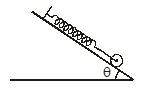Work done by Diff type of forces
Question

# A sphere of mass m, attached at its center to a spring on incline as shown in figure, is held in unstretched position of spring. Suddenly the sphere is set free, the maximum extension of spring is (friction is enough to prevent slipping)Moderate
Solution

## Maximum elongation $\frac{1}{2}{\mathrm{kx}}^{2}$ = mg sin θ.x by conservation of energy$\mathrm{x}=\frac{2\mathrm{mgsin\theta }}{\mathrm{k}}$

Get Instant Solutions